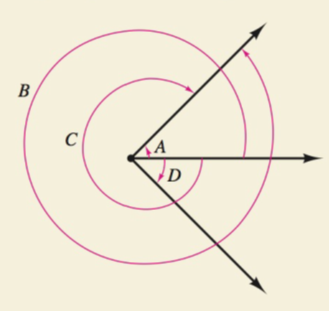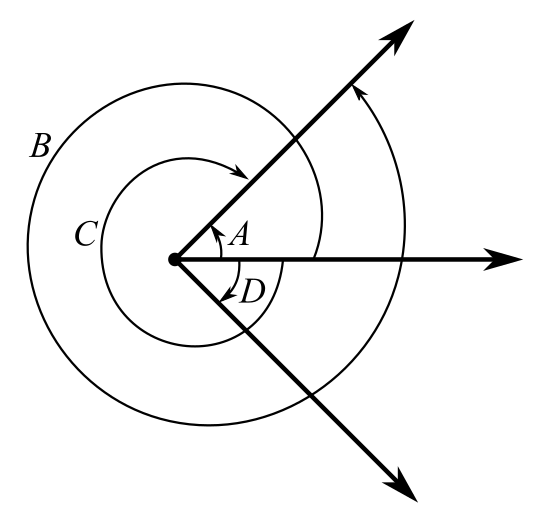Chapter 8.1, Problem 52E### Calculus: An Applied Approach (Min...

10th Edition
Ron Larson
ISBN: 9781305860919

#### Solutions

Chapter
Section### Calculus: An Applied Approach (Min...

10th Edition
Ron Larson
ISBN: 9781305860919
Textbook Problem
6 views

# How do you see it? Determine which angles in the figure are coterminal angles with angle A. Explain your reasoning.To determine

The angles which are coterminal angles with angle A for the provided figure. Also give explanation.Explanation

Given Information:

The figure provided below shows the angles in the circle as,

The figure provided below shows the initial and terminal sides.

From observation of the figure, the angle B and angle A have same initial side and same terminal sides.

So, the angle B is coterminal angle with angle A

### Still sussing out bartleby?

Check out a sample textbook solution.

See a sample solution

#### The Solution to Your Study Problems

Bartleby provides explanations to thousands of textbook problems written by our experts, many with advanced degrees!

Get Started

#### In Exercises 1520, simplify the expression. 17. 16x5yz481xyz54; x 0, y 0, z 0

Applied Calculus for the Managerial, Life, and Social Sciences: A Brief Approach

#### In Problems 1-6 simplify each fraction. 4.

Mathematical Applications for the Management, Life, and Social Sciences

#### Find mRST if mRT:mTS=1:4

Elementary Geometry for College Students

#### True or False: converges mean exists.

Study Guide for Stewart's Multivariable Calculus, 8th

#### True or False: If f(x) is continuous and decreasing, f(n) = an for all n = 1, 2, 3, …, and

Study Guide for Stewart's Single Variable Calculus: Early Transcendentals, 8th